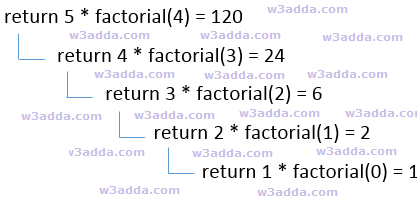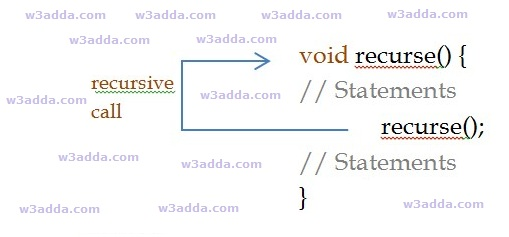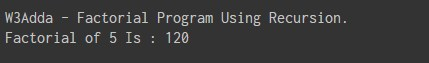# Dart Recursion

In this tutorial you will learn about the Dart Recursion and its application with practical example.

## What Is Recursion?

Recursion is the process where a function calls itself as its subroutine in order to solve a complex iterative task by dividing it into sub tasks. Any function which calls itself recursively is called recursive function, and the process of calling a function by itself is called recursion. Recursion leads to several number of iterative calls to the same function, however, it is important to have a base case to terminate the recursion.

Recursion is an efficient approach to solve a complex mathematical computation task by dividing it into sub tasks. This approach of solving a problem is called as Divide and Conquer.

Any problem that can be solved recursively, can also be solved iteratively but recursion is considered as more efficient method of programming as it requires the least amount of code to perform same complex task. Although recursion is not recommended for all problems, but it is best suited for some problems like sorting, searching, Inorder/Preorder/Postorder Tree Traversals, DFS of Graph algorithms. However, recursion must be implemented carefully, otherwise it may lead to an infinite loop if no base condition is met that will terminate the function.

Note :- Recursive function must have a valid terminating condition or base case, otherwise it will leads to an infinite loop.

## How recursion works?

To understand how recursion works lets have one of the popular example of recursion. In this example we will calculate the factorial of n numbers. The factorial of n numbers is expressed as a series of repetitive multiplication as shown below:

Example :-

dsfsdf## Dart Recursive Function

A recursive function is not much different from any other function, basically a function calling itself directly or indirectly is known as recursive function. A recursive function repeats itself several times, in order to compute or return final output. Recursive functions are quite common in computer programing as they allow programmers to write efficient programs with minimal code.

## Characteristics of a Recursive function

• A recursive function is a function which calls itself.
• The speed of a recursive program is slower because of stack overheads.
• A recursive function must have terminating conditions or base case, and recursive expressions.

Below is general syntax of a recursive function –

Syntax:-

Example:-## Dart Factorial Program Using Recursion

Example:-

Output:-In this tutorial we have learn about the Dart Recursion and its application with practical example. I hope you will like this tutorial.